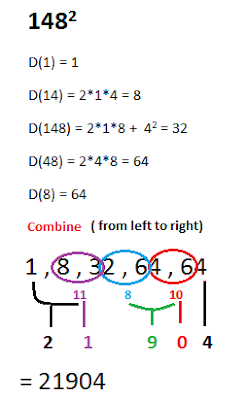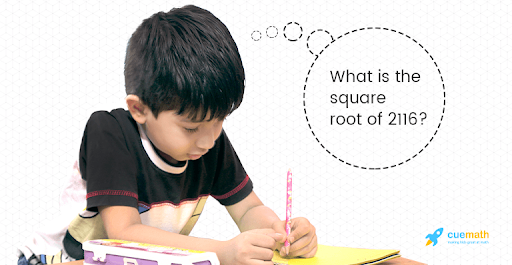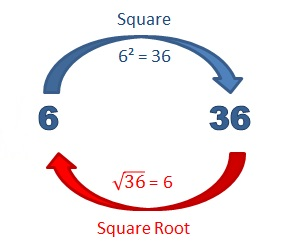Math Concepts
Squaring and Square Roots
54.9k views

 1 Introduction 2 Some simple Vedic Maths Tricks 3 Squaring Tricks! Square numbers faster 4 Square root Tricks! Learn how to find square roots 4 Summary 4 FAQs

12 November  2020

##Do you know how to square big numbers?

Do you find it hard or take too long to square numbers?

Bharthi Krishna Tritha, an Indian monk, wrote the book "VEDIC MATHEMATICS", which has a list of mathematical tricks to solve maths calculations faster than traditional methods. It gives the easiest way to solve addition, subtraction, multiplication, division, and related problems. Vedic mathematics can applied to pneumatics, statics, astronomy, and the financial domain.
Here we will learn about how to find the square root of a number.

If you ever want to read it again as many times as you want, here is a downloadable PDF to explore more.

## Some simple Vedic Maths Tricks

Tricks to Subtract any number from 10000, 1000 and 100
Everyone finds it difficult to subtract numbers from 10000 and 100.here is a simple technique stated in Vedic mathematics, subtract all numbers from 9 and last number by 10.

 Number :1000 - 589 Steps 9 - 5 9 - 8 10 - 9 Subtract the first two numbers 5 and 8 by 9 and the last number 9 by 10 Answer = 411

### Tricks to Multiply 3 digit numbers

 Number: 208 × 206 Steps 208 - 8 = 200 206 - 6 = 200 1.Subtract the number in units place by the number itself 206 + 8 = 214 2.Select number in units place among  given two number and add it to another number 214 × 200 = 42800 3.Multiply the above-obtained number with the number obtained in step 1. 8 × 6 = 48 4.Multiply the numbers in units place of the given question 42800 + 48 = 42848 5.add the answers from step 1 and Step 4. This is the answer. Answer: 42848

### Trick to Divide any large number with 5

 Number: 275 ÷ 5 Steps 275 × 2 = 550 1.Multiply the given number with 2 55.0 or 55 2.Move one decimal point Answer: 55

## Squaring Tricks! Square numbers faster

A number, when multiplied by the number itself the product obtained, is called "SQUARE OF THAT NUMBER ". Here are some examples followed by Square tricks.

 Example
 Number Square of the number 62 6 × 6 = 36 82 8 × 8 = 64 122 12 × 12 = 144

Now we shall learn few basic Vedic mathematics methods or Vedic Maths Squaring Tricks:

### Type 1: Squaring of number ending with 5.

Sutra is  "BY ONE MORE THAN PREVIOUS ONE"

Step 1: Add 1 to the first digit from the left and multiply by the number itself.
Step 2: Add 52 (25) at the end to the number obtained from step 1.

 Number Steps 65 6 × (6 + 1)  = 6 × 7 = 42 Add 1 to the left number (6) and multiply by the number itself and =4225 Add 52 (25) at the last of 42 Answer : 652 = 65 × 65 = 4225 85 8 × (8 + 1)  = 8 × 9 = 72 Add 1 to the left number (8) and multiply by the number itself and = 7225 add 52 (25) at last of 72 Answer : 852 = 85 × 85 = 7225 155 15 × (15 + 1)  = 15 × 16 = 240 Add 1 to the left number (15) and multiply by the number itself and = 24025 add 52 (25) at last of 240 Answer : 1552 = 155 × 155 = 2402

### Type 2: Squaring of numbers less than 50 and numbers not ending with 5.

 Number Steps 34 50 - 16 = 34 52 = 25 = 25 + (-16)  = 9 Square the first digit (5) of first part (50) then add part (-16) 162 = 256 Square the second part of number (16) 9 + 256 = 1156 Add the answers got in step 1 (9)and step 2 (256) Answer : 342 = 34 × 34 = 1156 28 50 - 22 = 28 52 = 25 = 25 + (-22)  = 3 Square the first digit (5) of first part (50) then add second part (-22) 222 = 484 Square the second part of number (22) 3 + 484 = 784 Add the answers got in step 1 (3)and step 2 (484) Answer : 282 = 28 × 28 = 784

### Type 3: Squaring of numbers less than 50 and numbers not ending with 5.

 Number Steps 74 50 + 24 = 74 52 = 25 Square the first digit (5) of first part (50) then add = 25 + 24 = 49 second part (24) 242 = 576 Square the second part of number 24 49 + 576 = 5476 Add the answers got in step 1 (49)and step 2 (576) Answer : 742 = 74 × 74 = 5476 57 50 + 7 = 57 52 = 25 = 25 + 7 = 32 Square the first digit (5) of first part (50) then add second part 7 72 = 49 Square the second part of number 7 32 + 49 = 3249 Add the answers got in step 1 (32)and step 2 (49) Answer : 572 = 57 × 57 = 3249

### Type 4: Squaring of number near to their base 10,100,1000, and so on:

 Number Steps 105 100 + 5 = Divide the given number to their base and number 105 + 5 = 110 Add the second part of number 5 to the given number (105) 52 = 25 Square the second part of the 52 11025 Combine the numbers from step 1 and step 2 Answer : 1052 = 105 × 105 = 11025 986 1000 - 986 = 14 986 - 14 = 972 The given number 986 is less than 14 from its base value 1000, so the deficient number 14 should be subtracted by the given number 986 142 = 196 Square of deficient number 211 972196 Combine the numbers from step 1 and step 2 Answer : 9862 = 986 × 986 = 972196
• If the number is lesser than its nearest base number then the deficient number is reduced from the given number.
• If the given number is greater than its nearest base number then the surplus number is added to the given number.

### Type 5: Squaring of a number near to their sub  base:

 Number Steps 306 300 + 6 = Divide the given number to their  sub base  and number 3 × (306 + 6)  = 3 ×312 = 936 Add the second part of number 6 to the given number (306) and multiply it by 3 62 = 36 Square the second part of the 62 93636 Combine the numbers from step 1 and step 2 Answer : 3062 = 306 × 306 = 93636 480 500 - 480 = 20 480 - 20= 5 ×(480 -20) =5 × 460 = 2300 The given number 480 is less than 20 from its sub base value 500, so the deficient number 20 should be subtracted by the given number 480 and multiplied by 5 202 =400 Square of deficient number 211 230400 Combine the numbers from step 1 and step 2 Answer : 4802 = 480 × 480 = 230400

### GENERAL METHOD TO FIND SQUARE OF NUMBER OR DUPLEX METHOD :

Duplex combination or Dwanda yoga is term used in terms for squaring and multiplication,denoted as D. Following are the basic methods used in duplex:

• D (a) = a2
• D (ab) = 2(ab)
• D (abc) = 2 (ac) + b2
• D (abcd) = 2 (ad) + 2(bc)
• D (abcde) = 2 (ae) + 2(bd) + c2
 Example## Square root Tricks! Learn how to find square roots

The square root of a number is the inverse operation of square of number.

 Example
 Number Square of the number Square root of the number 62 6 × 6 = 36 \begin{align}\sqrt {36} = 6\end{align} 82 8 × 8 = 64 \begin{align}\sqrt {64} = 8\end{align} 122 12 × 12 = 144 \begin{align}\sqrt {144} = 12\end{align}Points to be remembered before finding the square root of any number :

1. The given number is arranged as a group of two numbers from right to left. If a single number has remained at the left, then it is also considered as one group.
2. The number of groups derived for the given number will be the number of digits of the square root of that number.

Example : \begin{align}\sqrt {25} = 5\end{align}, here there are two digits so one group. So, the square root of 25 is 5.
\begin{align}\sqrt {169} = 13\end{align}, the number forms two groups (1, 69), so the square root is two-digit number 13.
3. If the square of a number is an odd number, then the square root is also an odd number, and if the square is an even number, then the square root is also an even number.
4.

 Number Square 12 1 22 4 32 9 42 16 52 25 62 36 72 49 82 64 92 81

 Logic to find the square root Observing the square of numbers from 1 to 9, we can analyze that an exact square ends with 1,4,5, 6,9, and 0 square root. These numbers will be rational numbers. If the square of the number ends with 2,3,7 and 8 then its square root will be an irrational number.

 Square Number ending with following number Square root ends with following number 1 1 or  9 4 2 or 8 5 5 6 4 or 6 9 3 or 7

Sutra is  "FIRST BY THE FIRST AND LAST BY THE LAST"

Let's solve few examples and learn how this technique works :

### Type 1: Square root of perfect square number:

 Number:\begin{align}\sqrt {3969}\end{align} Step 39 | 69 Grouping: from right to left 38 is greater than 36, the perfect square number of  62, and 69 is lesser than 64, which is a perfect square number of 82 3600 | 6400     602  |  802 Therefore the given number lies between 3600 and 6400 that means there square number will be between 60 and 80 So the first number in the square root will be 6 The number in the units places is 9 so the square number should be ending with 3 or 7 (as discussed above) The square root of the given number should be either 63 or 67 Applying digit sum method to find the square root 63 = 3969 (6 + 3) = 3+9+6+9    9  = 2 + 7      9 = 9 So the square root of number 3969 is 63 Answer : 3969 = 63

### Type 2: Square root of imperfect square number :

 Number Steps \begin{align}\sqrt {2}\end{align} The given number is not a perfect square 1 + 1 = 2. or 4 - 2 = 2 The nearest square number is 1 and 4 1 + 1/2 1 + 0.5 1.5 Square root of 1 is 1, add (1 ÷ [ 1 + 1]) i.e 1 divide by twice the perfect square number 1.5 The same when calculated in calculator gives 1.414 which is approximately equal to 1.5 \begin{align}\sqrt {20}\end{align} \begin{align}\sqrt {2}\end{align} 25 - 5 = 20 25 is the perfect square of 5 subtract it from (1/10) 1 divide by twice the perfect square 25 - 5/10 5 - 0.5 4.5 The same when calculated in calculator gives 4.47 which is approximately equal to 4.5

## Summary

After learning a few of the tricks to find square and square roots of the number, one will be able to easily find the square numbers and their square roots without any help from calculators. These tricks are very helpful in solving aptitude problems related to square and square roots in competitive exams.

Written by Nethravati C, Cuemath Teacher

Cuemath, a student-friendly mathematics and coding platform, conducts regular Online Classes for academics and skill-development, and their Mental Math App, on both iOS and Android, is a one-stop solution for kids to develop multiple skills. Understand the Cuemath Fee structure and sign up for a free trial.

## What is the square of a number?

If a number is multiplied by the same number itself then it is called a square of the number.
Example: square of 6 = 62
= 6 × 6 = 36

## What is the square root of a number?

A number which when multiplied  by the number itself gives a square of that number the inverse operation of this is known as the square root of the number.
Example \begin{align}\sqrt {25}=5\end{align}

## How to find the square root of a number?

Step 1 : group the given number as two digits in one group from right to left.
Step 2 : find the nearest perfect square number of the grouped numbers and analyse between which numbers the given number lies.
Step 3 : depending on the number in units place decide which number will be the possible square number
Step 4 : apply the digit sum method to find the possible square number.

## How to find the square of a number?

Square of a number can be determined by multiplying the number by the same number .
Example: 162  = 16 × 16 = 256

## What is the square root of 5?

The nearest perfect square number is 4, so 5 can be written has
\begin{align}\sqrt {5}=\sqrt {4}+1\end{align}
Adding two times of 4 i.e 8 to the denominator of second part
\begin{align}\sqrt {5}=\sqrt {4}+1/8\end{align}
Simplifying
\begin{align}\sqrt {5}=2 + 0.125\end{align}

\begin{align}\sqrt {5}= 2.125\end{align}

## What is the square root of 3?

The nearest perfect square number is 4, so 3 can be written has
\begin{align}\sqrt {3}=\sqrt {4}-1\end{align}
Adding two times of 4 i.e 8 to the denominator of second part
\begin{align}\sqrt {3}=\sqrt {4}-1/8\end{align}
On simplifying
\begin{align}\sqrt {3}=2 - 0.125\end{align}
\begin{align}\sqrt {3} = 1.875\end{align}

## What is the square root of 8?

The nearest perfect square number is 9, so 8 6can be written has
\begin{align}\sqrt {8} = \sqrt {9}-1\end{align}
Adding two times of 9 i.e 18 to the denominator of second part
\begin{align}\sqrt {8} = \sqrt {9}-1/18\end{align}
On simplifying
\begin{align}\sqrt {8} = 3 - 0.055 \end{align}
\begin{align}\sqrt {8} = 2.945 \end{align}

## What is the square root of 2?

The nearest perfect square number is 1, so 2 can be written has
\begin{align}\sqrt {2} = \sqrt {1}+1\end{align}
Adding two times of 1 i.e 2 to the denominator of second part
\begin{align}\sqrt {2} = \sqrt {1}+1/2\end{align}

On simplifying
\begin{align}\sqrt {2} = 1 + 0.5 \end{align}
\begin{align}\sqrt {2} = 1.5\end{align}

Related Articles